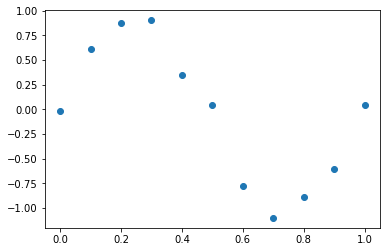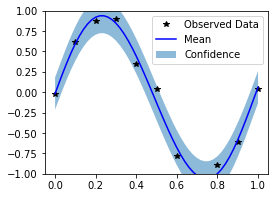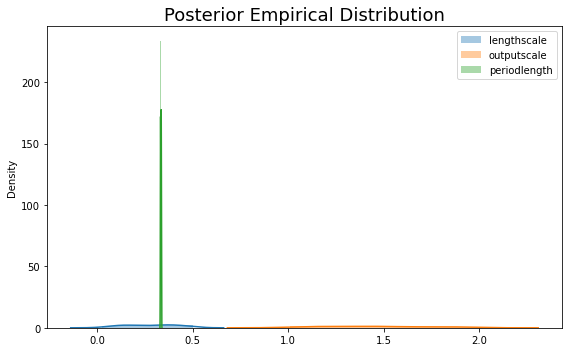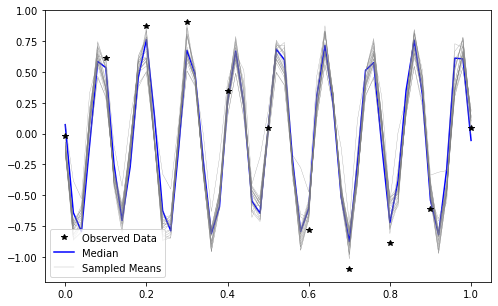# Gaussian process

## Gaussian Process Regression (with GPytorch)​

This tutorial assumes familiarity with the following:

1. Bean Machine modeling and inference
2. Gaussian Processes
3. GPyTorch

A Gaussian Process (GP) is a stochastic process commonly used in Bayesian non-parametrics, whose finite collection of random variables follow a multivariate Gaussian distribution. GPs are fully defined by a mean and covariance function:

$f\sim\mathcal{GP}\left(\mu(x),\mathbf{K}_f(x, x')\right)$

where $x,x'\in\mathbf{X}$ are two data points (e.g.) train and test), $\mu$ is the mean function (usually taken to be zero or constant), and $\mathbf{K}_f$ is the kernel function, which computes a covariance given two data points and a distance metric.

The aim is then to fit a posterior over functions. GPs allow us to learn a distribution over functions given our observed data and predict unseen data with well-calibrated uncertainty, and is commonly used in Bayesian Optimization as a surrogate function to maximize an objective. For a thorough introduction to Gaussian processes, please see 

With a PPL such as Bean Machine, we can be Bayesian about the parameters we care about, i.e. learn posterior distributions over these parameters rather than a point estimate.

# Install Bean Machine in Colab if using Colab.import sysif "google.colab" in sys.modules and "beanmachine" not in sys.modules:    !pip install beanmachine
import copyimport mathimport osimport warningsfrom functools import partialimport arviz as azimport beanmachineimport beanmachine.ppl as bmimport beanmachine.ppl.experimental.gp as bgpimport gpytorchimport matplotlib.pyplot as pltimport seaborn as snsimport torchimport torch.distributions as distfrom beanmachine.ppl.experimental.gp.models import SimpleGPfrom gpytorch.distributions import MultivariateNormalfrom gpytorch.kernels import Kernelfrom IPython.display import Markdown

The next cell includes convenient configuration settings to improve the notebook presentation as well as setting a manual seed for reproducibility.

# Eliminate excess UserWarnings from Python.warnings.filterwarnings("ignore")# Manual seedtorch.manual_seed(123)# Other settings for the notebook.smoke_test = "SANDCASTLE_NEXUS" in os.environ or "CI" in os.environ# Tool versionsprint("pytorch version: ", torch.__version__)print("gpytorch version: ", gpytorch.__version__)
Out:
pytorch version:  1.11.0gpytorch version:  1.6.0

Let's use some simple cyclic data:

x_train = torch.linspace(0, 1, 11)y_train = torch.sin(x_train * (2 * math.pi)) + torch.randn(x_train.shape) * 0.2x_test = torch.linspace(0, 1, 51).unsqueeze(-1)with torch.no_grad():    plt.scatter(x_train.numpy(), y_train.numpy())    plt.show()Since this data has a periodic trend to it, we will use a Periodic Kernel:

$k(x,x')=\sigma^2\exp\Big(-\frac{2}{\ell}\sin^2\Big(\pi\frac{|x-x'|}{p}\Big)\Big)$

where $p$, $\ell$, $\sigma^2$ are the periodicity, length scale, and output scale of the function respectively, the (hyper)parameters of the kernel we want to learn.

## MAP Estimation (with GPyTorch)​

GPytorch's exact inference algorithms allow you to compute maximum a posteriori (MAP) estimates of kernel parameters. Since a SimpleGP extends a GPytorch ExactGP model, you can use GPytorch to optimize the model. Let's try that, closely following the GPytorch regression tutorial.

class Regression(SimpleGP):    def __init__(self, x_train, y_train, mean, kernel, likelihood, *args, **kwargs):        super().__init__(x_train, y_train, mean, kernel, likelihood)    def forward(self, data, batch_shape=()):        """        Computes the GP prior given data. This method should always        return a torch.distributions.MultivariateNormal        """        shape = data.shape[len(batch_shape)]        jitter = torch.eye(shape, shape) * 1e-5        for _ in range(len(batch_shape)):            jitter = jitter.unsqueeze(0)        if isinstance(self.mean, gpytorch.means.Mean):            # demo using gpytorch for MAP estimation            mean = self.mean(data)        else:            # use Bean Machine for learning posteriors            if self.training:                mean = self.mean(batch_shape).expand(data.shape[len(batch_shape) :])            else:                mean = self.mean.expand(data.shape[:-1])  # overridden for evaluation        cov = self.kernel(data) + jitter        return MultivariateNormal(mean, cov)
kernel = gpytorch.kernels.ScaleKernel(base_kernel=gpytorch.kernels.PeriodicKernel())likelihood = gpytorch.likelihoods.GaussianLikelihood()mean = gpytorch.means.ConstantMean()gp = Regression(x_train, y_train, mean, kernel, likelihood)
optimizer = torch.optim.Adam(    gp.parameters(), lr=0.1)  # Includes GaussianLikelihood parametersmll = gpytorch.mlls.ExactMarginalLogLikelihood(likelihood, gp)gp.eval()  # this converts the BM model into a gpytorch modelnum_iters = 1 if smoke_test else 100for i in range(num_iters):    optimizer.zero_grad()    output = gp(x_train)    loss = -mll(output, y_train)    loss.backward()    if i % 10 == 0:        print(            "Iter %d/%d - Loss: %.3f"            % (                i + 1,                100,                loss.item(),            )        )    optimizer.step()
Out:
Iter 1/100 - Loss: 1.082Iter 11/100 - Loss: 0.504Iter 21/100 - Loss: 0.040Iter 31/100 - Loss: -0.385Iter 41/100 - Loss: -0.755Iter 51/100 - Loss: -0.939Iter 61/100 - Loss: -0.947Iter 71/100 - Loss: -0.966Iter 81/100 - Loss: -0.960Iter 91/100 - Loss: -0.954
with torch.no_grad():    observed_pred = likelihood(gp(x_test))    # Initialize plot    f, ax = plt.subplots(1, 1, figsize=(4, 3))    # Get upper and lower confidence bounds    lower, upper = observed_pred.confidence_region()    # Plot training data as black stars    ax.plot(x_train.numpy(), y_train.numpy(), "k*")    # Plot predictive means as blue line    ax.plot(x_test.squeeze().numpy(), observed_pred.mean.numpy(), "b")    # Shade between the lower and upper confidence bounds    ax.fill_between(x_test.squeeze().numpy(), lower.numpy(), upper.numpy(), alpha=0.5)    ax.set_ylim([-1, 1])    ax.legend(["Observed Data", "Mean", "Confidence"])Not bad! Our GP fits this simple function fairly well. However, we've only captured data uncertainty, not parameter uncertainty. It can often be the case that calibrating parameter uncertainty may lead to better predictive performance. In the next section, we'll do just that using Bean Machine's NUTS algorithm.

## Fully Bayesian Inference with Bean Machine​

Let's reuse the same model, but this time, use Bean Machine to learn posteriors over the parameters. In train mode, SimpleGP is a simple wrapper around gpytorch.models.ExactGP that lifts learnable parameters to BM random variables. Next, lets define our parameters $p$, $\sigma^2$, and $\ell$ in addition to mean and observation noise as random variables with the priors they are sampled from.

@bm.random_variabledef outputscale():    return dist.Uniform(torch.tensor(1.0), torch.tensor(2.0))@bm.random_variabledef lengthscale():    return dist.Uniform(torch.tensor([0.01]), torch.tensor([0.5]))@bm.random_variabledef period_length():    return dist.Uniform(torch.tensor([0.05]), torch.tensor([2.5]))@bm.random_variabledef noise():    return dist.Uniform(torch.tensor([0.05]), torch.tensor([0.3]))@bm.random_variabledef mean(batch_shape=()):    batch_shape += (1,)    a = -1 * torch.ones(batch_shape)    b = torch.ones(batch_shape)    return dist.Uniform(a, b)

Similarly, we'll create kernel and likelihood objects, this time passing our random variables as the hyperparameters. Note that the kernels are beanmachine.kernels instead of gpytorch.kernels.

kernel = bgp.kernels.ScaleKernel(    base_kernel=bgp.kernels.PeriodicKernel(        period_length_prior=period_length, lengthscale_prior=lengthscale    ),    outputscale_prior=outputscale,)likelihood = bgp.likelihoods.GaussianLikelihood(noise_prior=noise)gp = Regression(x_train, y_train, mean, kernel, likelihood)

Now we can run inference as we would with any other Bean Machine model.

num_samples = 1 if smoke_test else 100num_adaptive_samples = 0 if smoke_test else num_samples // 2num_chains = 1 if smoke_test else 2# bind the data to the forward call so it can be invoked in the likelihoodqueries = [mean(), lengthscale(), period_length(), outputscale(), noise()]gp_prior = partial(gp, x_train)obs = {gp.likelihood(gp_prior): y_train}nuts = bm.SingleSiteNoUTurnSampler()samples = nuts.infer(    queries=queries,    observations=obs,    num_samples=num_samples,    num_adaptive_samples=num_adaptive_samples,    num_chains=num_chains,)
Out:
Samples collected:   0%|          | 0/150 [00:00<?, ?it/s]Samples collected:   0%|          | 0/150 [00:00<?, ?it/s]

Let's take a look at how our model fit. We will plot the samples of our posterior as well as the predictives generated from our GP.

summary_df = az.summary(samples.to_inference_data())Markdown(summary_df.to_markdown())
meansdhdi_3%hdi_97%mcse_meanmcse_sdess_bulkess_tailr_hat
period_length()0.3840.0530.3290.4480.0360.0293172.1
mean()0.1130.624-0.8420.9920.0720.08369481.05
noise()0.1630.0780.0570.2990.0090.00758221.03
outputscale()1.4320.281.0441.9260.0320.02372861.01
lengthscale()0.2240.1470.0170.4770.040.02912161.13
lengthscale_samples = samples.get_chain(0)[lengthscale()]outputscale_samples = samples.get_chain(0)[outputscale()]period_length_samples = samples.get_chain(0)[period_length()]mean_samples = samples.get_chain(0)[mean()]noise_samples = samples.get_chain(0)[noise()]
if not smoke_test:    plt.figure(figsize=(8, 5))    sns.distplot(lengthscale_samples, label="lengthscale")    sns.distplot(outputscale_samples, label="outputscale")    sns.distplot(period_length_samples[: int(num_samples / 2)], label="periodlength")    plt.legend()    plt.title("Posterior Empirical Distribution", fontsize=18)    plt.tight_layout()    plt.show()To generate predictions, we will convert our model to a Gpytorch model by running in eval mode. We load our posterior samples with a python dict, keyed on the parameter namespace and valued on the torch tensor of samples. Note the unsqueezes to allow broadcasting of the data dimension to the right.

gp.eval()  # converts to Gpytorch model in eval modegp.bm_load_samples(    {        "kernel.outputscale": outputscale_samples,        "kernel.base_kernel.lengthscale": lengthscale_samples.unsqueeze(-1),        "kernel.base_kernel.period_length": period_length_samples.unsqueeze(-1),        "likelihood.noise": noise_samples,        "mean": mean_samples,    })expanded_x_test = x_test.unsqueeze(0).repeat(num_samples, 1, 1)output = gp(expanded_x_test.detach(), batch_shape=(num_samples,))

Now we let's plot a few predictive samples from our GP. As you can see, we can draw different kernels, each of which paramaterizes a Multivariate Normal.

if not smoke_test:    with torch.no_grad():        f, ax = plt.subplots(1, 1, figsize=(8, 5))        ax.plot(x_train.numpy(), y_train.numpy(), "k*", zorder=10)        ax.plot(            x_test.numpy(),            output.mean.median(0).detach().numpy(),            "b",            linewidth=1.5,        )        for i in range(min(20, num_samples)):            ax.plot(                x_test.numpy(),                output.mean[i].detach().numpy(),                "gray",                linewidth=0.3,                alpha=0.8,            )        ax.legend(["Observed Data", "Median", "Sampled Means"])Rasmussen, Carl and Williams, Christopher. Gaussian Processes for Machine Learning. 2006.